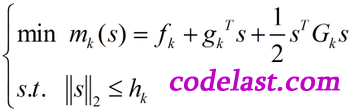# [原创] LM(Levenberg-Marquard)算法的实现

LM算法，全称为Levenberg-Marquard算法，它可用于解决非线性最小二乘问题，多用于曲线拟合等场合。

LM算法的实现并不算难，它的关键是用模型函数 $f$ 对待估参数向量 $p$ 在其邻域内做线性近似，忽略掉二阶以上的导数项，从而转化为线性最小二乘问题，它具有收敛速度快等优点。LM算法属于一种“信赖域法”——所谓的信赖域法，此处稍微解释一下：在最优化算法中，都是要求一个函数的极小值，每一步迭代中，都要求目标函数值是下降的，而信赖域法，顾名思义，就是从初始点开始，先假设一个可以信赖的最大位移 $s$ ，然后在以当前点为中心，以 $s$ 为半径的区域内，通过寻找目标函数的一个近似函数（二次的）的最优点，来求解得到真正的位移。在得到了位移之后，再计算目标函数值，如果其使目标函数值的下降满足了一定条件，那么就说明这个位移是可靠的，则继续按此规则迭代计算下去；如果其不能使目标函数值的下降满足一定的条件，则应减小信赖域的范围，再重新求解。

LM算法需要对每一个待估参数求偏导，所以，如果你的目标函数 $f$ 非常复杂，或者待估参数相当地多，那么可能不适合使用LM算法，而可以选择Powell算法——Powell算法不需要求导。$({G_k} + \mu I)s = - {g_k}$ 确定 ${s_k}$ 表征。

$\mu \ge 0$ ，使得 ${G_k} + \mu I$ 正定，并解线性方程组 $({G_k} + \mu I){s_k} = - {g_k}$ 求出 ${s_k}$

• 从初始点 ${x_0}$ ${\mu _0} > 0$ 开始迭代
• 到第 $k$ 步时，计算 ${x_k}$ ${\mu _k}$
• 分解矩阵 ${G_k} + {\mu _k}I$ ，若不正定，令 ${\mu _k} = 4{\mu _k}$ 并重复到正定为止
• 解线性方程组 $({G_k} + {\mu _k}I){s_k} = - {g_k}$ 求出 ${s_k}$ 并计算 ${r_k}$
• ${r_k} < 0.25$ ，令 ${\mu _{k + 1}} = 4{\mu _k}$ ；若 ${r_k} > 0.75$ ，令 ${\mu _{k + 1}} = \frac{{{\mu _k}}}{2}$ ；若 $0.25 \le {r_k} \le 0.75$ ，令 ${\mu _{k + 1}} = {\mu _k}$
• ${r_k} \le 0$ ，说明函数值是向着上升而非下降的趋势变化了（与最优化的目标相反），这说明这一步走错了，而且错得“离谱”，此时，不应该走到下一点，而应“原地踏步”，即 ${x_{k + 1}} = {x_k}$ ，并且和上面 ${r_k} < 0.25$ 的情况一样对 ${\mu _k}$ 进行处理。反之，在 ${r_k} > 0$ 的情况下，都可以走到下一点，即 ${x_{k + 1}} = {x_k} + {s_k}$
• 迭代的终止条件： $\left\| {{g_k}} \right\| < \varepsilon$ ，其中 $\varepsilon$ 是一个指定的小正数（大家可以想像一下二维平面上的寻优过程（函数图像类似于抛物线），当接近极小值点时，迭代点的梯度趋于0）

➤➤ 版权声明 ➤➤### 《[原创] LM(Levenberg-Marquard)算法的实现》有7条评论

1. 非常感谢指点，现在我正在使用C#编写LM算法，能否告知你那个时候是用什么语言编写的lm代码吗？谢谢了！

回复
• 哦，这样啊，你还有代码吗？能给我传一份吗？谢谢了，我的邮箱是389454688@qq.com，谢谢了！！！！

回复
• 抱歉，涉及到版权，不能发给你。不过网上到处都有此类代码下载，例如：http://www.ics.forth.gr/~lourakis/levmar/